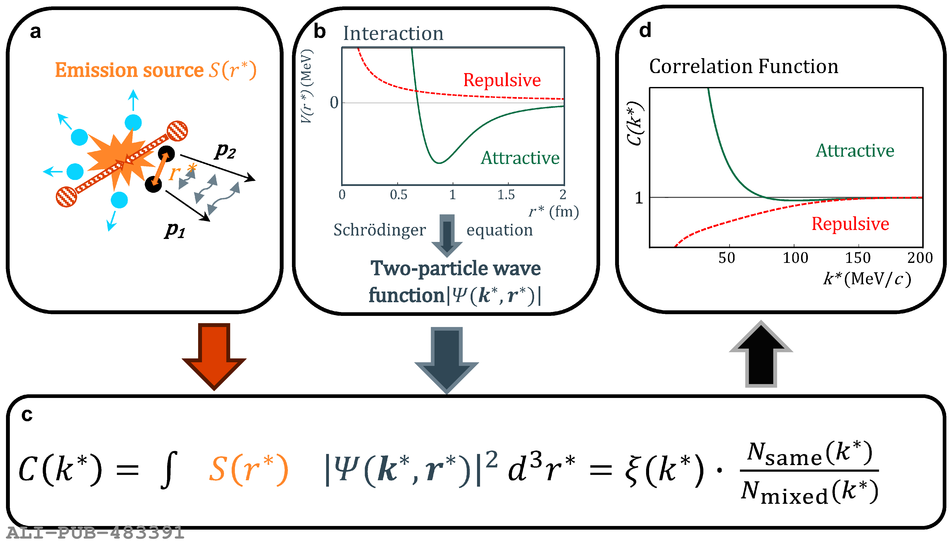# Figure 1

 Schematic representation of the correlation method. a, A collision of two protons generates a particle source $S(\rs)$ from which a hadron--hadron pair with momenta $\bm{p}\bf{_1}$ and $\bm{p}\bf{_2}$ emerges at a relative distance $r^*$ and can undergo final-state interaction before being detected Consequently, the relative momentum \ks is either reduced or increased via an attractive or a repulsive interaction, respectively b, Example of attractive (green) and repulsive (dotted red) interaction potentials, V(\rs), between two hadrons, as a function of their relative distance Given a certain potential, a non-relativistic Schr\"odinger equation is used to obtain the corresponding two-particle wave function, $\psi(\bm{k}^*,\bm{r}^*)$ c, The equation of the calculated (second term) and measured (third term) correlation function < em>C< /em>(\ks), where $N_{\mathrm{same}}(\ks)$ and $N_{\mathrm{mixed}}(\ks)$ represent the \ks distributions of hadron--hadron pairs produced in the same and in different collisions, respectively, and $\xi(\ks)$ denotes the corrections for experimental effects d, Sketch of the resulting shape of < em>C< /em>(\ks) The value of the correlation function is proportional to the interaction strength It is above unity for an attractive (green) potential, and between zero and unity for a repulsive (dotted red) potential